```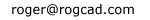----------------

home

Balloting

Simple trig

Logistic map

The Attractor of Henon

Number doubling

Barnsley's Fern

The Sierpinski Triangle

Double a number

Choose an initial value between 0 and 1.
Double it.  Drop the integer part.  Repeat.
Values occur in discreet bunches.  Bunches
occur in multiples of five, depending on the
number of decimal places used.  Repeating
patterns occur within the bunches.

Do not select number = .5

Use 4 or 5 digit numbers.

(Complete program code for VisualBASIC
and DOS BASIC at bottom of page)

Graphed circular: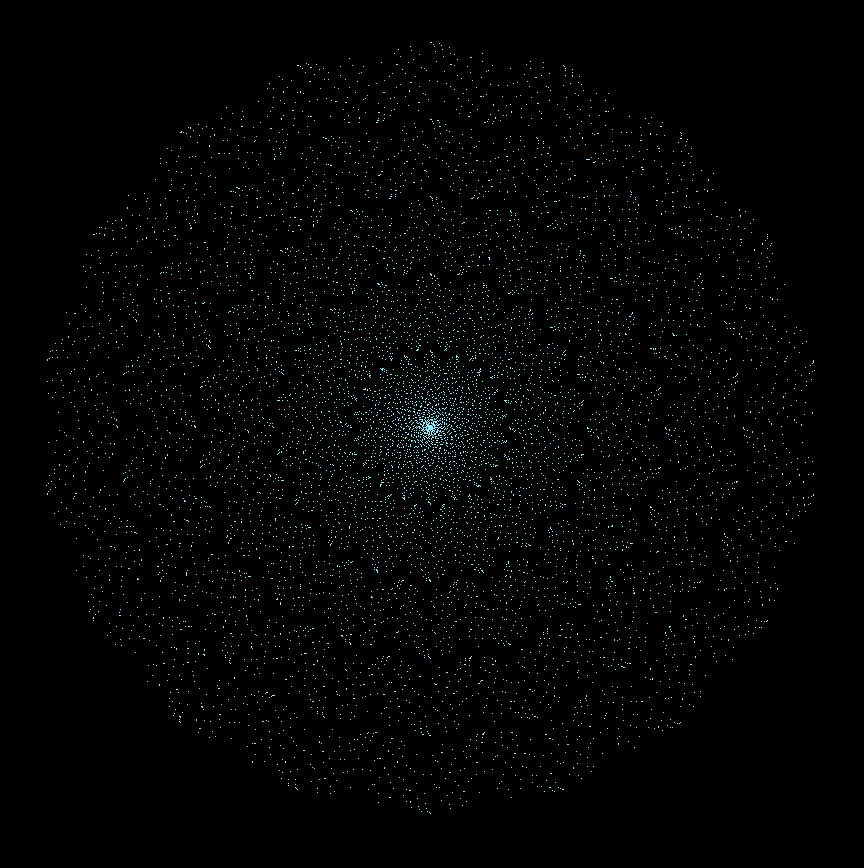Graphed straight:Here are four images from my DOSBASIC version: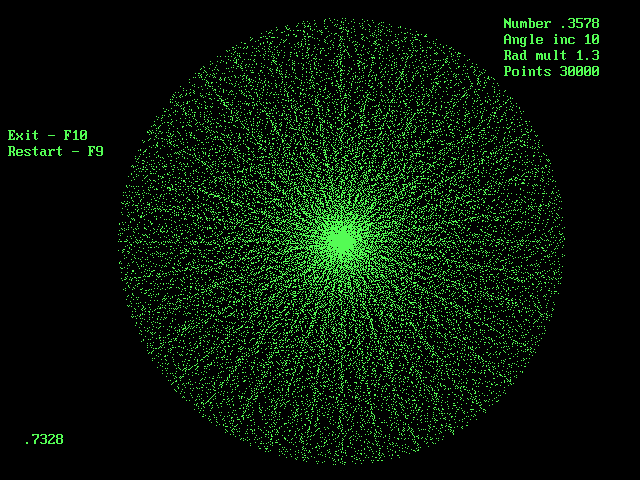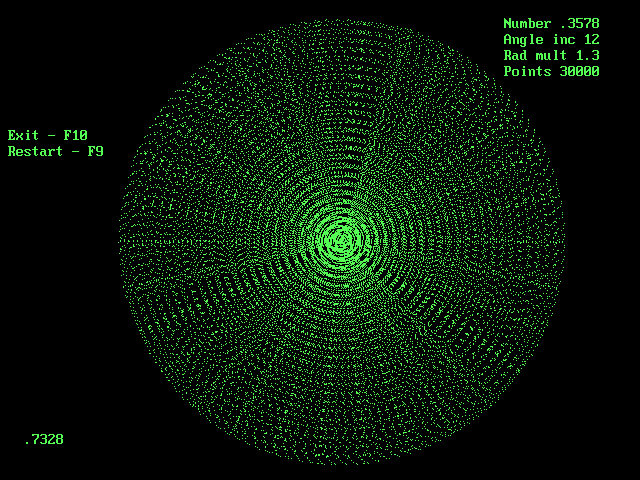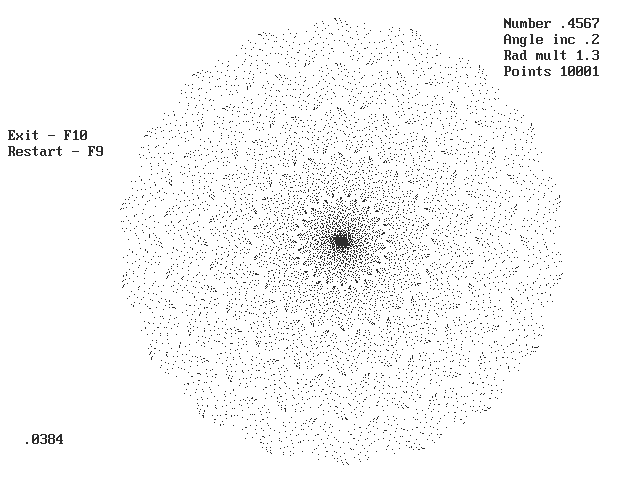VisualBASIC program code (circular):

Private Sub run_Click()

num = Val(numb.Text)  REM: not = .5
ITER = Val(itera.Text)
anginc = Val(angleinc.Text) * (3.14159265358979 / 180)

For a = 1 To ITER

num = num * 2
If num > 1 Then num = num - 1

num = num * 50001      REM: Overrides VB rounding errors
num = Int(num)
num = num / 50000

angle = angle + anginc

X = num * (Cos(angle) * ra) + 430
Y = num * 1.2284 * (Sin(angle) * ra) + 530

viewport.PSet (X, Y), RGB(0, 0, 0)

Next a

finalnum.Text = "        "
finalnum.Text = Str\$(num)

End Sub

===========================================================

VisualBASIC program code (straight):

Private Sub dsrunbtn_Click()

trial = Val(trialsbox.Text)
amp = Val(dsamp.Text)
inc = Val(dsinc.Text)
num = Val(numb.Text)       REM: not = .5
ITER = Val(itera.Text)

For b = 1 To trial

X = 0

For a = 1 To ITER

num = num * 2
If num > 1 Then num = num - 1

num = num * 50001      REM: Overrides VB rounding errors
num = Int(num)
num = num / 50000

X = X + inc
Y = (num * amp) + 10

viewport.PSet (X, Y), RGB(0, 0, 0)

Next a

Next b

finalnum.Text = "        "
finalnum.Text = Str\$(num)

End Sub

===========================================================

DOS BASIC program code (circular):

SCREEN 12
CLEAR
CLS

5:

LOCATE 2, 3: PRINT "                             "
LOCATE 3, 3: PRINT "                             "
LOCATE 4, 3: PRINT "                             "
LOCATE 5, 3: PRINT "                             "

LOCATE 2, 64: PRINT "                       "
LOCATE 3, 64: PRINT "                       "
LOCATE 4, 64: PRINT "                       "
LOCATE 5, 64: PRINT "                       "

KEY(9) ON
ON KEY(9) GOSUB 400

KEY(10) ON
ON KEY(10) GOSUB 500

COLOR 10

NUM = 0 : ANGINC = 0 : RADMULT = 0 : POINTS = 0
X = 0 : Y = 0 : ANGLE = 0 : RAD = 0

LOCATE 2, 64: PRINT "Number"; NUMB
LOCATE 3, 64: PRINT "Angle inc"; ANGINCR
LOCATE 5, 64: PRINT "Points"; POINTSS

LOCATE 2, 3: INPUT "Number 0 - 1"; NUMB          REM: not = .5
LOCATE 3, 3: INPUT "angle increment"; ANGINCR
LOCATE 5, 3: INPUT "points"; POINTSS

NUM = NUMB
ANGINC = ANGINCR
POINTS = POINTSS

LOCATE 2, 3: PRINT "                             "
LOCATE 3, 3: PRINT "                             "
LOCATE 4, 3: PRINT "                             "
LOCATE 5, 3: PRINT "                             "

LOCATE 2, 64: PRINT "                       "
LOCATE 3, 64: PRINT "                       "
LOCATE 4, 64: PRINT "                       "
LOCATE 5, 64: PRINT "                       "

LOCATE 2, 64: PRINT "Number"; NUMB
LOCATE 3, 64: PRINT "Angle inc"; ANGINCR
LOCATE 5, 64: PRINT "Points"; POINTSS

ANGINC = ANGINC * (3.14159265358979 / 180)

LOCATE 8, 2: PRINT "Start - press spacebar"
LOCATE 9, 2: PRINT "Exit - F10
LOCATE 10, 2: PRINT "Restart - F9"

VIEW (1, 1)-(639, 479)
CLS

LOCATE 2, 64: PRINT "Number"; NUMB
LOCATE 3, 64: PRINT "Angle inc"; ANGINCR
LOCATE 5, 64: PRINT "Points"; POINTSS

40 I\$ = INKEY\$
IF I\$ = "" THEN 40

LOCATE 9, 2: PRINT "Exit - F10
LOCATE 10, 2: PRINT "Restart - F9"

LOCATE 8, 1: PRINT "                               "

FOR C = 1 TO POINTS

NUM = NUM * 2
IF NUM > 1 THEN NUM = NUM - 1

NUM = NUM * 50001      REM: Overrides QB rounding errors
NUM = INT(NUM)
NUM = NUM / 50000

ANGLE = ANGLE + ANGINC

X = NUM * 2 * (COS(ANGLE) * RAD) + 340
Y = NUM * 2 * (SIN(ANGLE) * RAD) + 240

PSET (X, Y)

NEXT C

LOCATE 28,3: PRINT "          "
LOCATE 28,3: PRINT STR\$(NUM)

50 I\$ = INKEY\$
IF I\$ = "" THEN 50

400 KEY(9) OFF : GOTO 5

500 KEY(10) OFF: KEY(9) OFF
END

==============================================================

home

Balloting

Simple trig

Logistic map

The Attractor of Henon

Number doubling

Barnsley's Fern

The Sierpinski Triangle

```